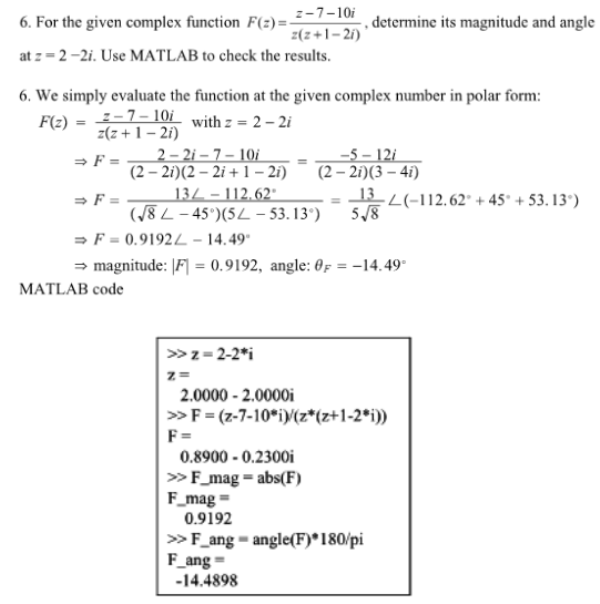For the given complex function F(z)=(z-7-10i)/(z(z+1-2i)), determine its magnitude and angle at z=2-2i. Use MATLAB to check the results.For the given complex function F(z)=(z-7-10i)/(z(z+1-2i)), determine its magnitude and angle at z=2-2i. Use MATLAB to check the results.

System Dynamics Page 2 dynamics dynamics dynamics dynamics dynamics dynamics dynamics System dynamics Page 3